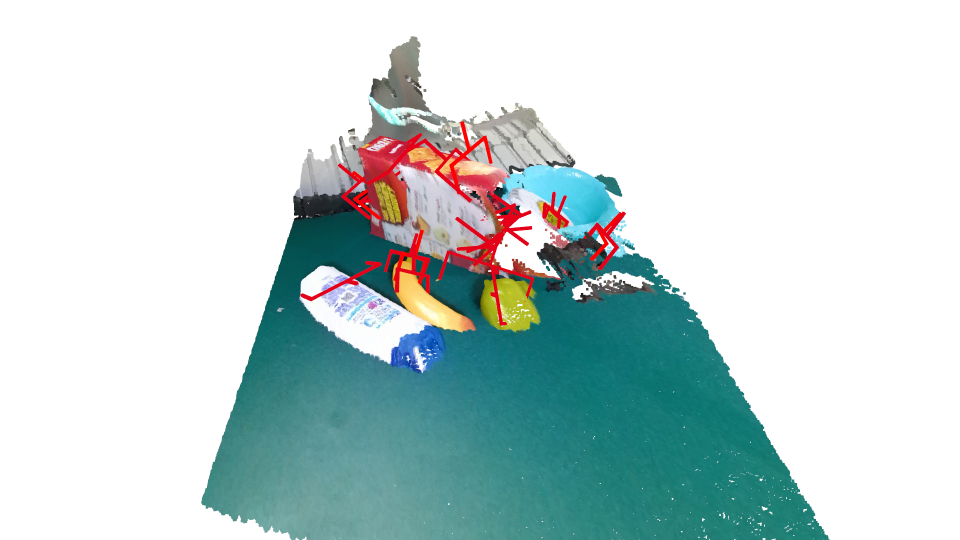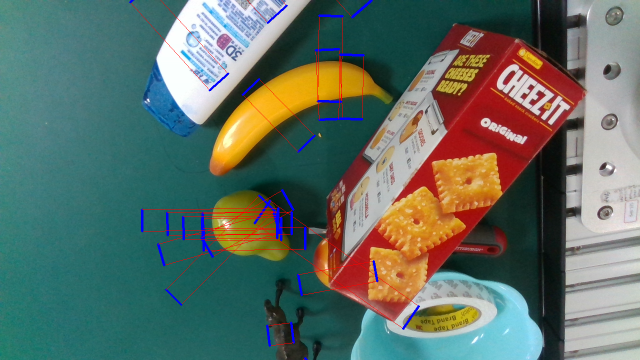Both 6d and rect format labels can be loaded.

First, import relative libs.

```from graspnetAPI import GraspNet
import open3d as o3d
import cv2

```

Then, get a GraspNet instance and setup parameters.

```####################################################################
graspnet_root = '/home/gmh/graspnet' # ROOT PATH FOR GRASPNET
####################################################################

sceneId = 1
annId = 3

# initialize a GraspNet instance
g = GraspNet(graspnet_root, camera='kinect', split='train')
```

Load GraspLabel in 6d format and visulize the result.

```# load grasps of scene 1 with annotation id = 3, camera = kinect and fric_coef_thresh = 0.2
_6d_grasp = g.loadGrasp(sceneId = sceneId, annId = annId, format = '6d', camera = 'kinect', fric_coef_thresh = 0.2)
print('6d grasp:\n{}'.format(_6d_grasp))

# visualize the grasps using open3d
geometries = []
geometries.append(g.loadScenePointCloud(sceneId = sceneId, annId = annId, camera = 'kinect'))
geometries += _6d_grasp.random_sample(numGrasp = 20).to_open3d_geometry_list()
o3d.visualization.draw_geometries(geometries)
```Load GraspLabel in rect format and visulize the result.

```# load rectangle grasps of scene 1 with annotation id = 3, camera = realsense and fric_coef_thresh = 0.2
rect_grasp = g.loadGrasp(sceneId = sceneId, annId = annId, format = 'rect', camera = 'realsense', fric_coef_thresh = 0.2)
print('rectangle grasp:\n{}'.format(rect_grasp))

# visualize the rectanglegrasps using opencv
bgr = g.loadBGR(sceneId = sceneId, annId = annId, camera = 'realsense')
img = rect_grasp.to_opencv_image(bgr, numGrasp = 20)
cv2.imshow('rectangle grasps', img)
cv2.waitKey(0)
cv2.destroyAllWindows()
```